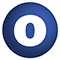disjunction

object disjunction extends Monoid[Boolean]

Source
AnyVal.scala
Linear Supertypes
Monoid[Boolean], Semigroup[Boolean], AnyRef, Any
Ordering
1. Alphabetic
2. By inheritance
Inherited
1. disjunction
2. Monoid
3. Semigroup
4. AnyRef
5. Any
1. Hide All
2. Show all
Visibility
1. Public
2. All

Type Members

1. trait MonoidLaw extends SemigroupLaw

Monoid instances must satisfy scalaz.Semigroup.SemigroupLaw and 2 additional laws:

2. trait SemigroupLaw extends AnyRef

A semigroup in type F must satisfy two laws:

Value Members

1. final def !=(arg0: AnyRef): Boolean

Definition Classes
AnyRef
2. final def !=(arg0: Any): Boolean

Definition Classes
Any
3. final def ##(): Int

Definition Classes
AnyRef → Any
4. final def ==(arg0: AnyRef): Boolean

Definition Classes
AnyRef
5. final def ==(arg0: Any): Boolean

Definition Classes
Any
6. def append(f1: Boolean, f2: ⇒ Boolean): Boolean

The binary operation to combine `f1` and `f2`.

The binary operation to combine `f1` and `f2`.

Implementations should not evaluate tbe by-name parameter `f2` if result can be determined by `f1`.

Definition Classes
disjunctionSemigroup
7. final def applicative: Applicative[[α]Boolean]

A monoidal applicative functor, that implements `point` and `ap` with the operations `zero` and `append` respectively.

A monoidal applicative functor, that implements `point` and `ap` with the operations `zero` and `append` respectively. Note that the type parameter `α` in `Applicative[({type λ[α]=F})#λ]` is discarded; it is a phantom type. As such, the functor cannot support scalaz.Bind.

Definition Classes
Monoid
8. final def apply: Apply[[α]Boolean]

An scalaz.Apply, that implements `ap` with `append`.

An scalaz.Apply, that implements `ap` with `append`. Note that the type parameter `α` in `Apply[({type λ[α]=F})#λ]` is discarded; it is a phantom type. As such, the functor cannot support scalaz.Bind.

Definition Classes
Semigroup
9. final def asInstanceOf[T0]: T0

Definition Classes
Any
10. final def category: Category[[α, β]Boolean]

Every `Monoid` gives rise to a scalaz.Category, for which the type parameters are phantoms.

Every `Monoid` gives rise to a scalaz.Category, for which the type parameters are phantoms.

Definition Classes
Monoid
Note

`category.monoid` = `this`

11. def clone(): AnyRef

Attributes
protected[java.lang]
Definition Classes
AnyRef
Annotations
@throws( ... )
12. final def compose: Compose[[α, β]Boolean]

Every `Semigroup` gives rise to a scalaz.Compose, for which the type parameters are phantoms.

Every `Semigroup` gives rise to a scalaz.Compose, for which the type parameters are phantoms.

Definition Classes
Semigroup
Note

`compose.semigroup` = `this`

13. final def eq(arg0: AnyRef): Boolean

Definition Classes
AnyRef
14. def equals(arg0: Any): Boolean

Definition Classes
AnyRef → Any
15. def finalize(): Unit

Attributes
protected[java.lang]
Definition Classes
AnyRef
Annotations
@throws( classOf[java.lang.Throwable] )
16. final def getClass(): Class[_]

Definition Classes
AnyRef → Any
17. def hashCode(): Int

Definition Classes
AnyRef → Any
18. final def ifEmpty[B](a: Boolean)(t: ⇒ B)(f: ⇒ B)(implicit eq: Equal[Boolean]): B

Definition Classes
Monoid
19. final def isInstanceOf[T0]: Boolean

Definition Classes
Any
20. def isMZero(a: Boolean)(implicit eq: Equal[Boolean]): Boolean

Whether `a` == `zero`.

Whether `a` == `zero`.

Definition Classes
Monoid
21. def monoidLaw: MonoidLaw

Definition Classes
Monoid
22. val monoidSyntax: MonoidSyntax[Boolean]

Definition Classes
Monoid
23. def multiply(value: Boolean, n: Int): Boolean

For `n = 0`, `zero` For `n = 1`, `append(zero, value)` For `n = 2`, `append(append(zero, value), value)`

For `n = 0`, `zero` For `n = 1`, `append(zero, value)` For `n = 2`, `append(append(zero, value), value)`

Definition Classes
Monoid
24. final def ne(arg0: AnyRef): Boolean

Definition Classes
AnyRef
25. final def notify(): Unit

Definition Classes
AnyRef
26. final def notifyAll(): Unit

Definition Classes
AnyRef
27. final def onEmpty[A, B](a: Boolean)(v: ⇒ B)(implicit eq: Equal[Boolean], mb: Monoid[B]): B

Definition Classes
Monoid
28. final def onNotEmpty[B](a: Boolean)(v: ⇒ B)(implicit eq: Equal[Boolean], mb: Monoid[B]): B

Definition Classes
Monoid
29. def semigroupLaw: SemigroupLaw

Definition Classes
Semigroup
30. val semigroupSyntax: SemigroupSyntax[Boolean]

Definition Classes
Semigroup
31. final def synchronized[T0](arg0: ⇒ T0): T0

Definition Classes
AnyRef
32. def toString(): String

Definition Classes
AnyRef → Any
33. final def wait(): Unit

Definition Classes
AnyRef
Annotations
@throws( ... )
34. final def wait(arg0: Long, arg1: Int): Unit

Definition Classes
AnyRef
Annotations
@throws( ... )
35. final def wait(arg0: Long): Unit

Definition Classes
AnyRef
Annotations
@throws( ... )
36. def zero: Boolean

The identity element for `append`.

The identity element for `append`.

Definition Classes
disjunctionMonoid